# 💯 A Level Past Papers H2 Math (9758) Trend (2010-2019) | Ingel Soong

Square
4.9/5 - 1 reviews

Looking for JC H2 tuition?

or  Ingel at 96726733 now to avoid disappointment!

# A Level H2 Maths (9758) Past Year Questions Trend

The following is a compilation of all the A Level H2 Mathematics marks allocation across the past ten years series (2010-2019).

Note Correlation and Linear Regression is not tested for A Level as part of the common last topic.

## Past Year Topic Trend Heat Map (Full Paper)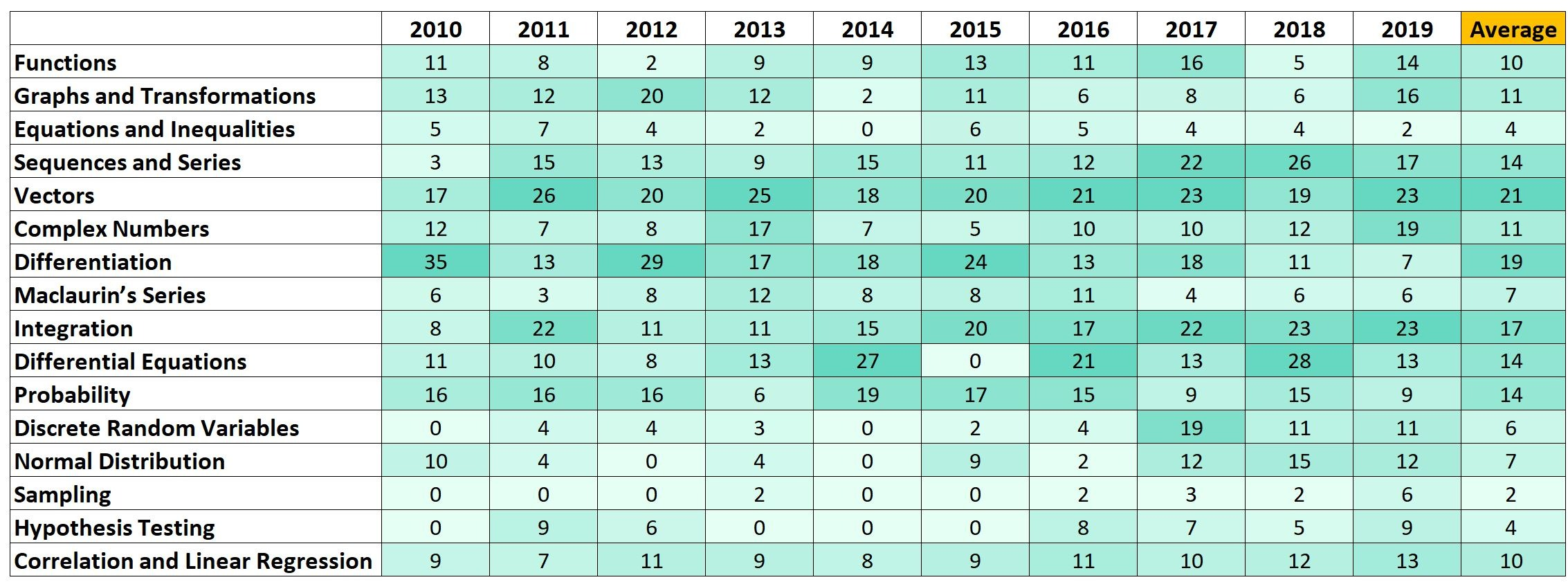Some observations can be made for the H2 Maths full paper:

• Vectors, differentiation and integration remains very popular topics to be tested across the years.
• Equations and inequalities, sampling and hypothesis testing are the least popular topics to be tested across the years.
• However, do note hypothesis testing is tested more frequently in recent years.
• Sequences and series, integration, discrete random variables, hypothesis testing seem to be tested more frequently in recent years.
• Differentiation seem to be tested less frequently in recent years, but remain a popular topic.

## Past Year Topic Trend Heat Map (Paper 1)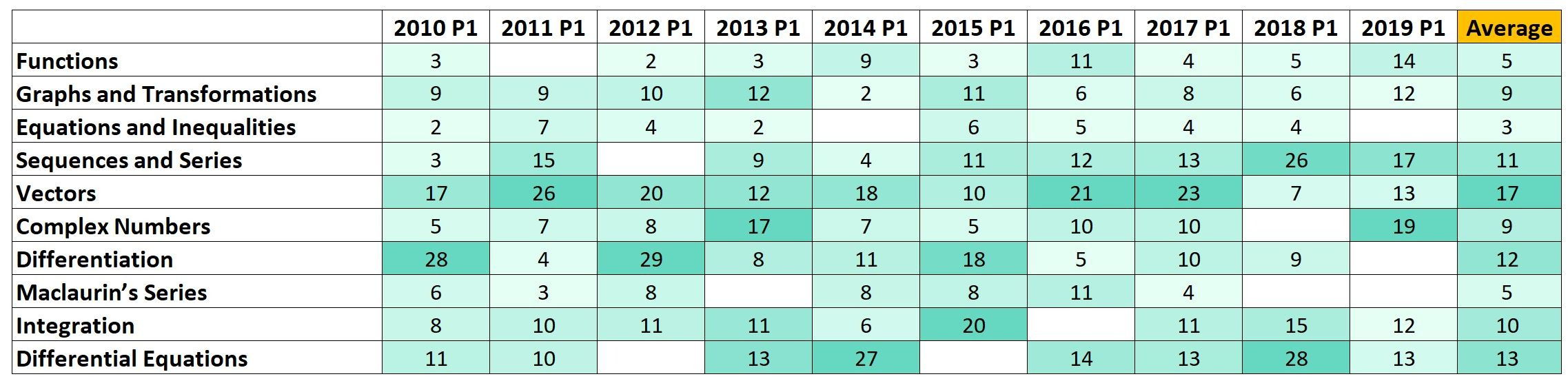Some observations can be made for H2 Maths paper 1:

• Vectors, differential equations and differentiation remains very popular topics to be tested across the years.
• Equations and inequalities, functions and Maclaurin’s series are the least popular topics to be tested across the years.
• However, do note functions is tested more frequently in recent years.
• Functions, sequences and series seem to be tested more frequently in recent years.
• Maclaurin’s series seem to be tested less frequently in recent years.

## Past Year Topic Trend Heat Map (Paper 2)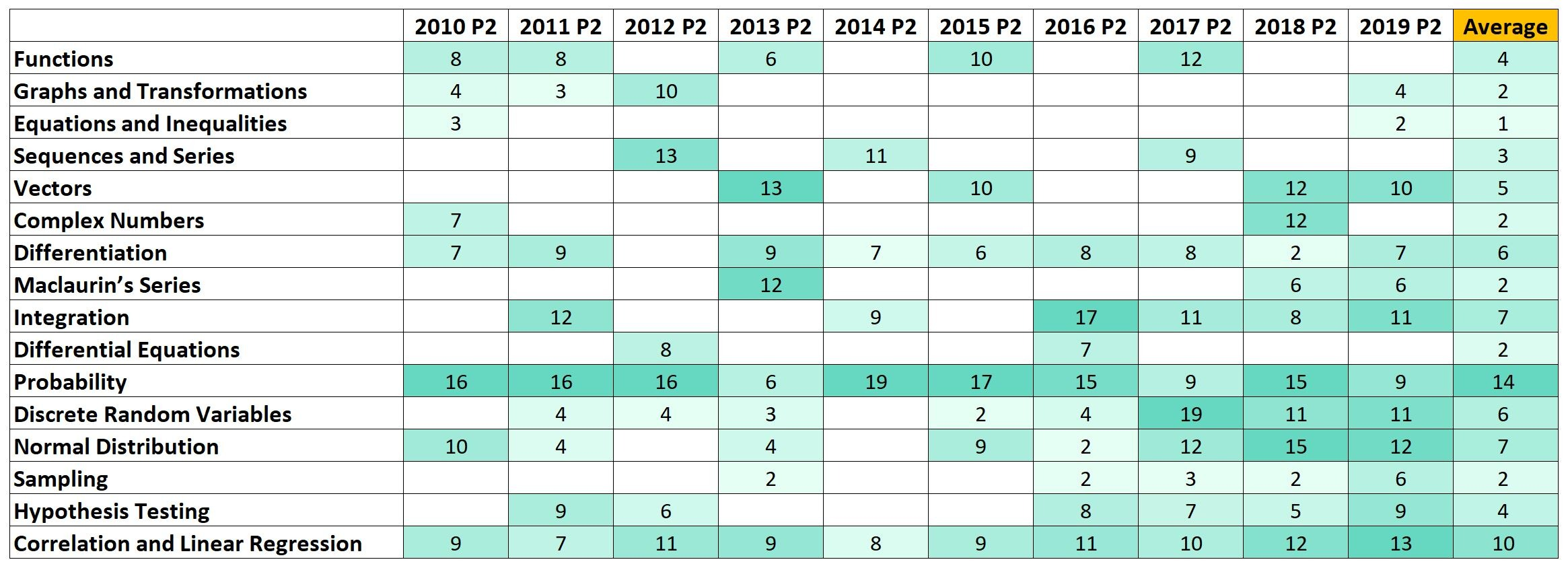Some observations can be made for H2 Maths paper 2:

• Apart from topics that are no longer in syllabus, probability, correlation and linear regression, integration remain very popular topics to be tested across the years.
• Differential equations, sampling, equations and inequalities are the least popular topics to be tested across the years.
• However, do note hypothesis testing is tested more frequently in recent years.
• Integration, discrete random variables, normal distribution, sampling and hypothesis testing seem to be tested more frequently in recent years.

## Past Year Topic Trend (Full Paper)

The following is the same yearly distribution of marks across the topics for H2 Maths full paper, presented in bar chart format.

Refer to above heat map for comments.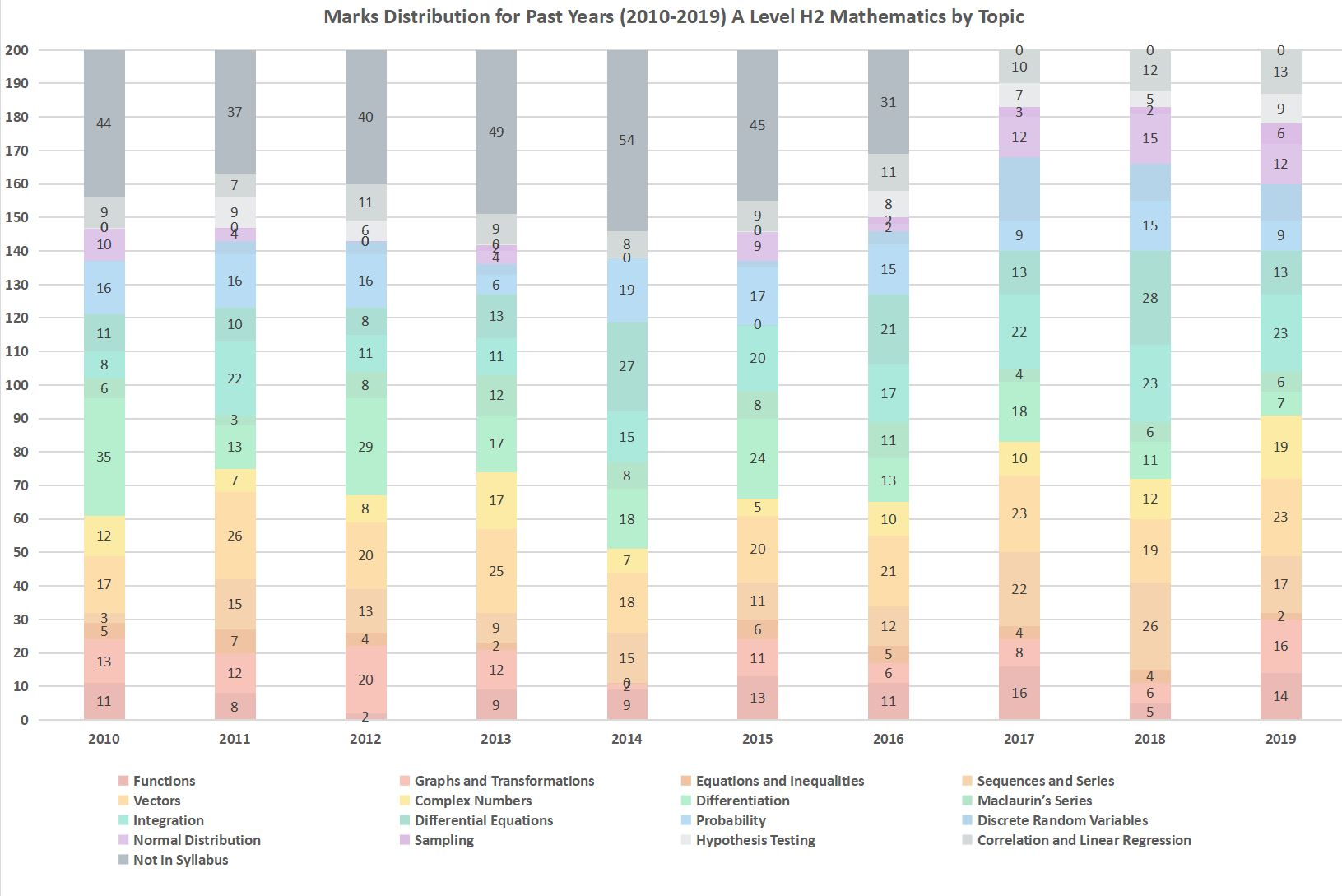## Past Year Topic Trend (Paper 1)

The following is the same yearly distribution of marks across the topics for H2 Maths paper 1, presented in bar chart format.

Refer to above heat map for comments.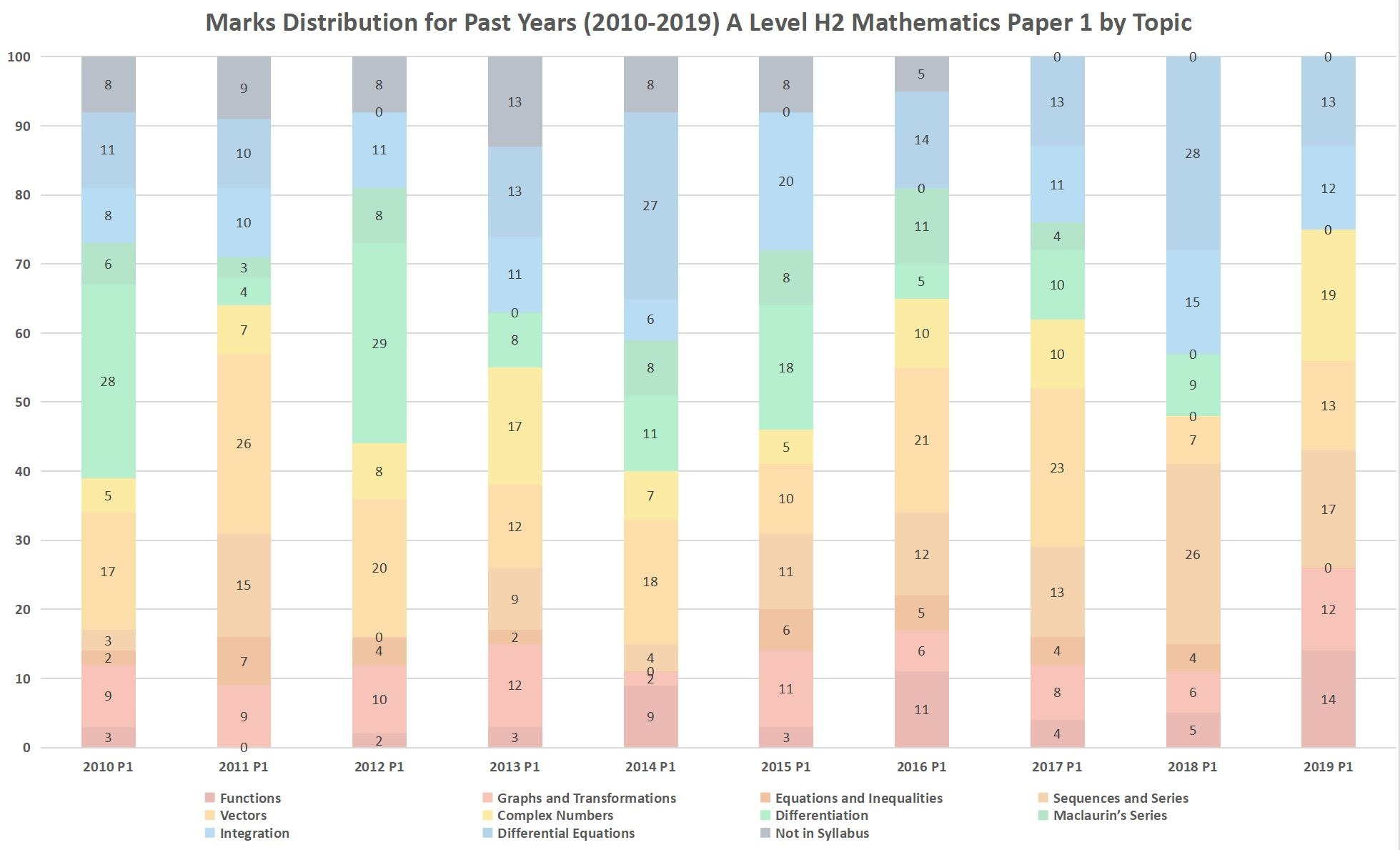## Past Year Topic Trend (Paper 2)

The following is the same yearly distribution of marks across the topics for H2 Maths paper 2, presented in bar chart format.

Refer to above heat map for comments.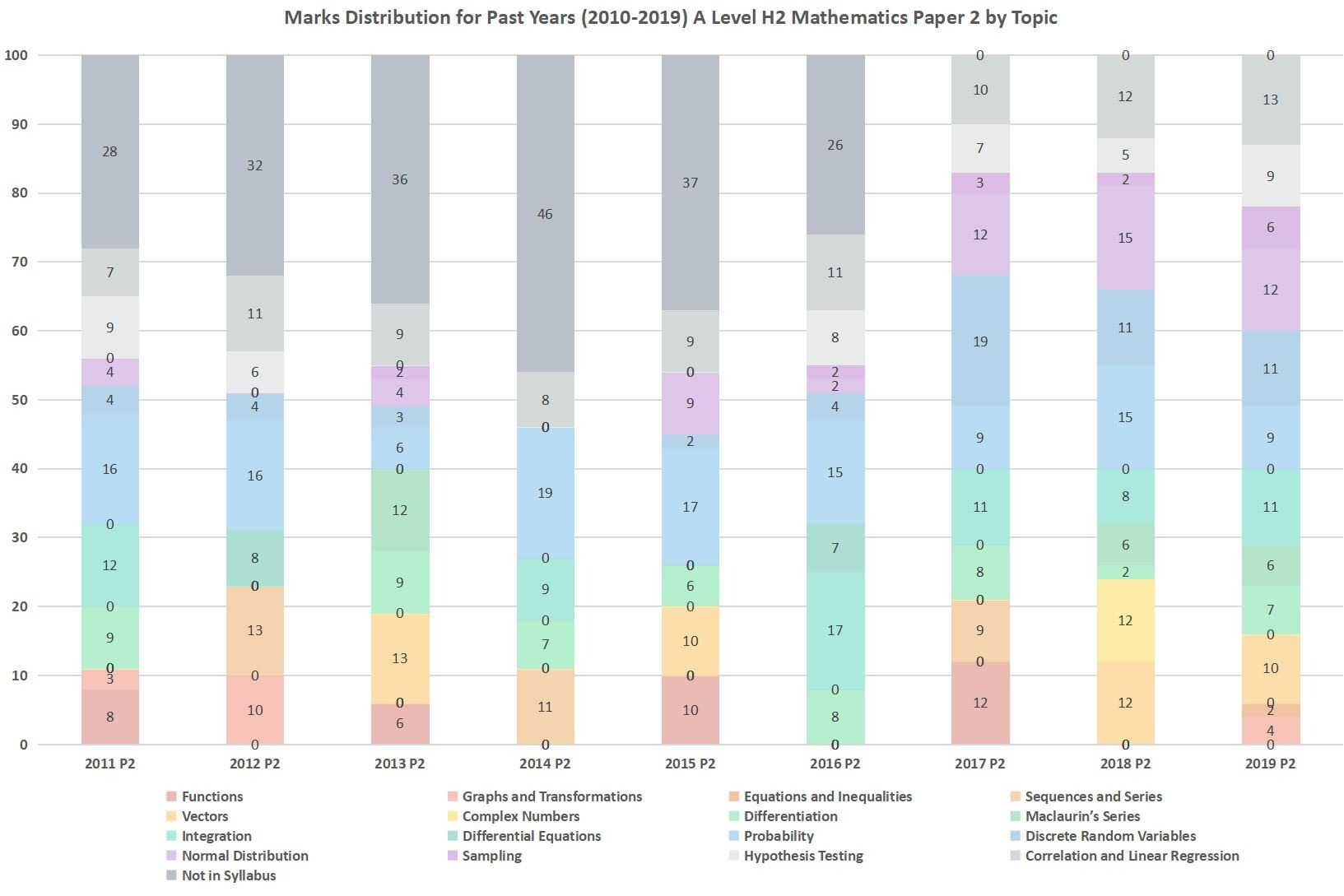## Popular Topics, Ranked (Paper 1)

The following is a ranking of popular paper 1 topics, average across ten years.

Some observations can be made for H2 Maths paper 1:

• Vectors, differential equations and differentiation remains very popular topics to be tested across the years.
• Barring topics meant for paper 2, equations and inequalities, functions and Maclaurin’s series are the least popular topics to be tested across the years.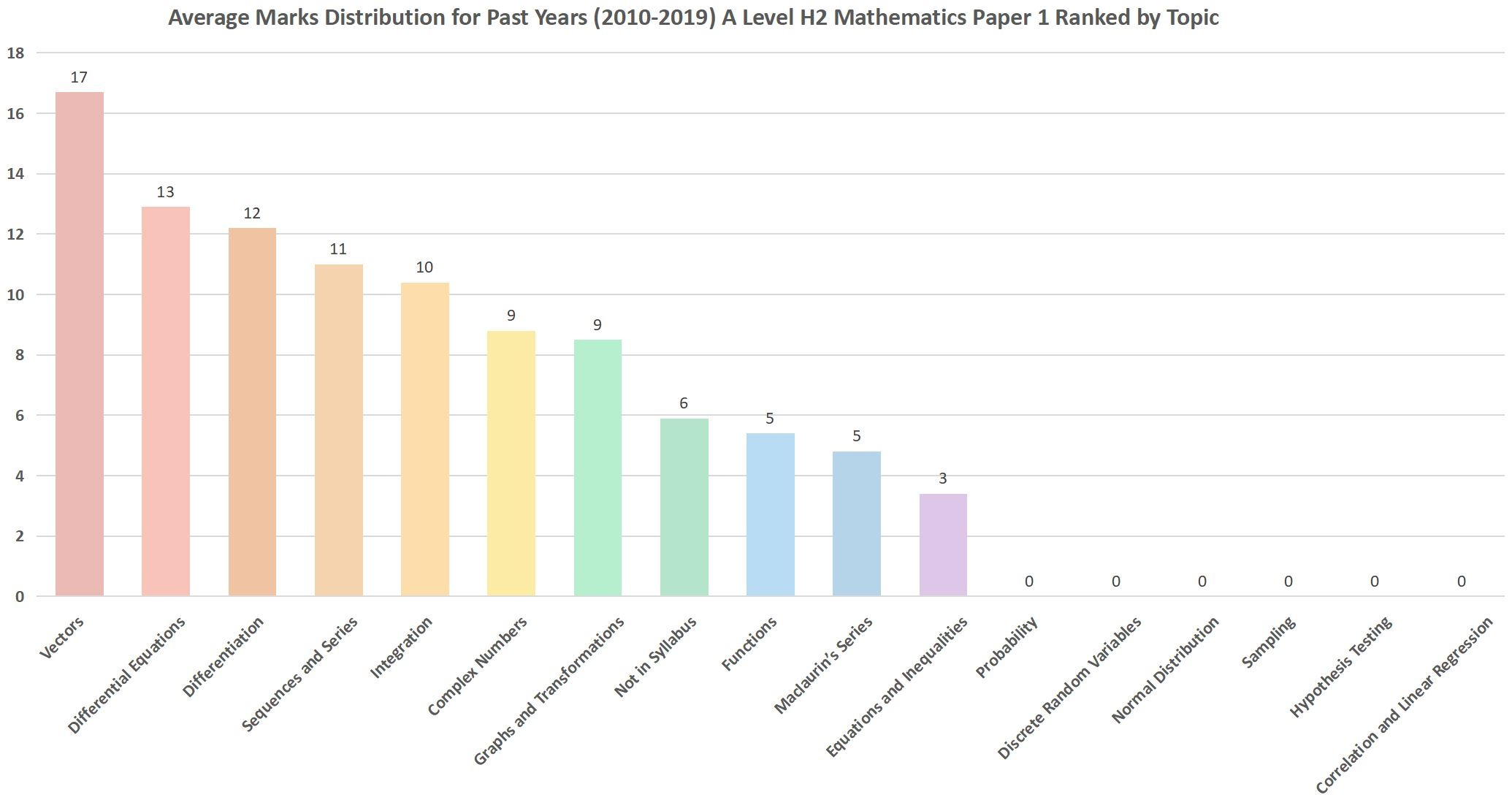## Popular Topics, Ranked (Paper 2)

The following is a ranking of popular paper 2 topics, average across ten years.

Some observations can be made for H2 Maths paper 2:

• Apart from topics that are no longer in syllabus, probability, correlation and linear regression, integration remain very popular topics to be tested across the years.
• Differential equations, sampling, equations and inequalities are the least popular topics to be tested across the years.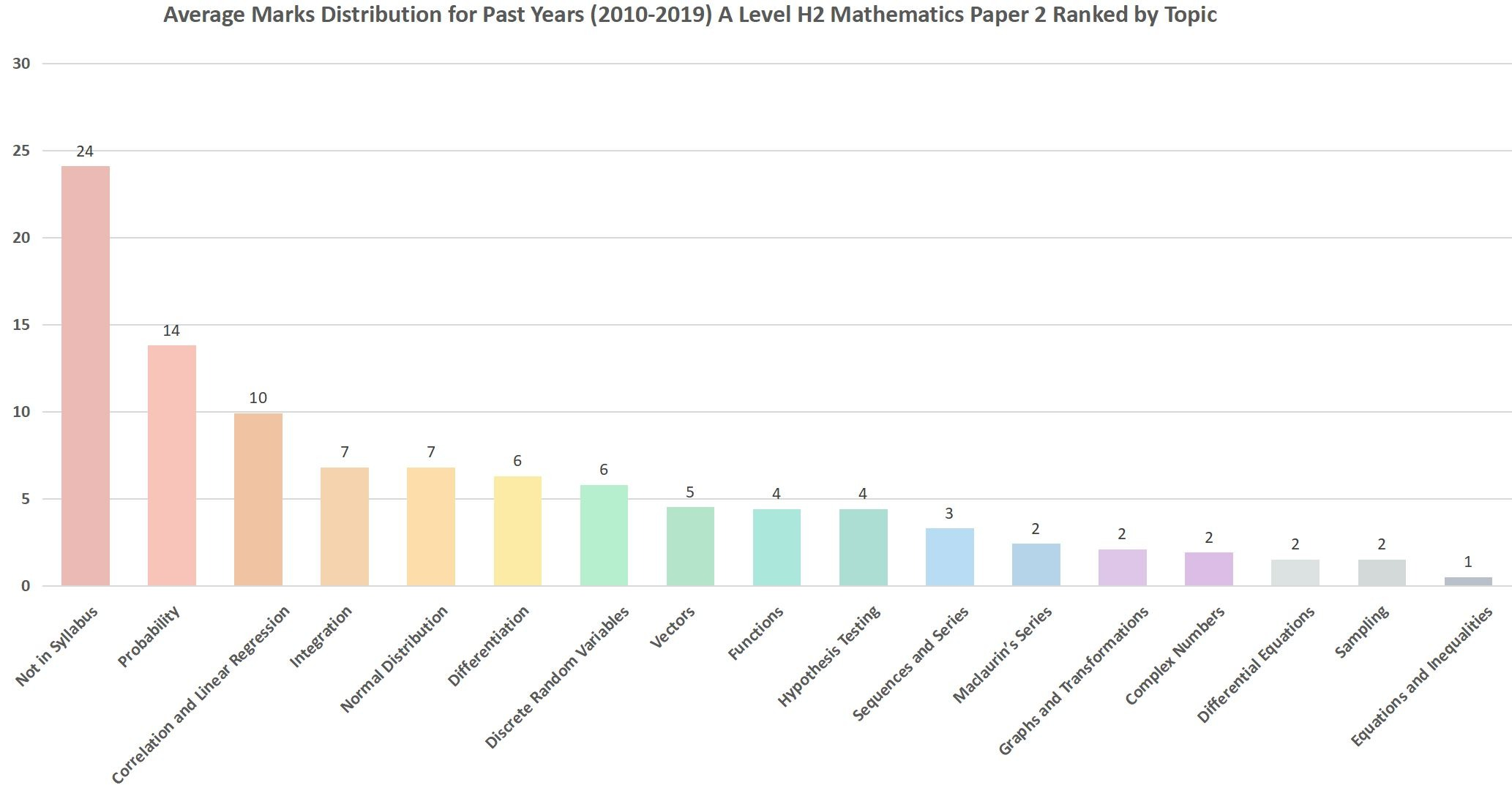Write in the comments below what do you think will be the hot topics for A Level H2 Maths in the year 2020.

Notes:

• As some questions can span multiple topics, discretion is made to segregate them to their respective topics.
• Many topics have been removed in recent years, such as mathematical induction, loci of complex numbers, Poisson distribution etc.
• This data is by no means indicative of this year’s examination questions.
• Errors may exist.
• Use with discretion.

Call or WhatsApp Ingel at 96726733 for a discussion of 2020 papers.